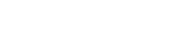# width

The desired width of the output image.

The `width` parameter enables dynamic width resizing based on pixels and percent values.

The `{value}` can be either a positive integer or a number less than 1. If the value is an integer greater than or equal to 1, the value is interpreted as a pixel width. If the width parameter is less than 1, the value is interpreted as a percentage width.

Unless a `height` parameter is present, the `width` value will cause the height of the image to be scaled in proportion to the requested width. If both width and height are omitted, the input image dimensions are used.

## Syntax

``width={value}``

`value` may be one of:

• Absolute width: An integer between 1 and 8192.
• Relative width: A fraction between 0 and 0.99 (e.g. ,`0.5`) or a percentage between 0 and 100 followed by the letter `p` (e.g., `50p`). In either case the value indicates the desired width relative to the image's natural width.
1. The maximum output dimensions are 8,192 x 8,192 pixels.
2. If the image is animated, the maximum output dimensions apply to the sum of the area of all the frames of the image. For example, a 1024x1024px GIF could have 64 frames before it would exceed the size limit.
3. Because we use a positive number less than 1 to return a percentage-based scaled image, in order to return an image that is 100% or more, a `p` suffix can be appended to the value. For example, `width=250p` would return an image that is `250%` the width of the original. We use `p` as a simple way to represent percent.
4. If the requested width is a fraction of a pixel (e.g., as a result of applying a percentage value for `width`), it is rounded to the nearest integer.

## Examples

Click the links to view the transformed image using a demo Fastly IO service.

Example usageDescription
`?width=200`Resize the width to 200px
`?width=0.10`Resize the width to 10% of the input image
`?width=150p`Resize the width to 150% of the input image
`?width=200&height=0.50`Force resize to a width of 200px and a height that is 50% of the original.
`?width=150&height=150&fit=bounds`Fit image within a 150 x 150px rectangle
`?width=200&dpr=2`Resize the width to 400px (200 CSS pixels for screens with a device pixel ratio of 2)

BETA